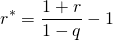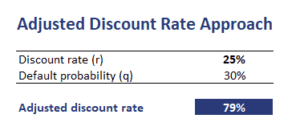In venture capital, the Adjusted Discount Rate Approach is a method to account for the higher risk in venture capital investing. As with most other valuation approaches, the discount rate used and the estimate of the terminal value will have a huge impact on the value we obtain for a company.

Very often, the owners of a start-up company are too optimistic about the prospects of a company. In particular, they grossly underestimate the likelihood that the company can fail. Many venture capital firms adjust for the higher risk and probability of failure by adjusting the discount rate.

On this page, we discuss one such approach. An Excel spreadsheet that implements the adjusted discount approach for venture capital investments is available at the bottom of the page.

Before we continue, we should mention that there is a second approach that is sometimes used by venture capital firms to adjust valuation estimates. This second approach consists of applying scenario analysis to come up with a more accurate terminal value estimate. The purpose of both the adjusted discount approach and scenario analysis is to place bounds on the value of the company before negotiating the terms of an investment.

Let’s adjust the discount rate. The following formula adjusts the discount rate to reflect the fact that the risk that the company may fail. In particular, we calculate r for the probability of failure qwhere r is the unadjusted discount rate and r* is the adjusted discount rate.

Let’s consider a very simple numerical example that implements the above formula. The table below reports the result. Clearly, simple spreadsheet software is enough to adjust the discount rate.## Summary

We discussed the adjusted discount rate approach to adjust the discount rate to reflect the fact that the firm may fail. Another method is to deflate each future cash flow for the cumulative probability that the company will fail. The method we presented, however, is more straightforward.